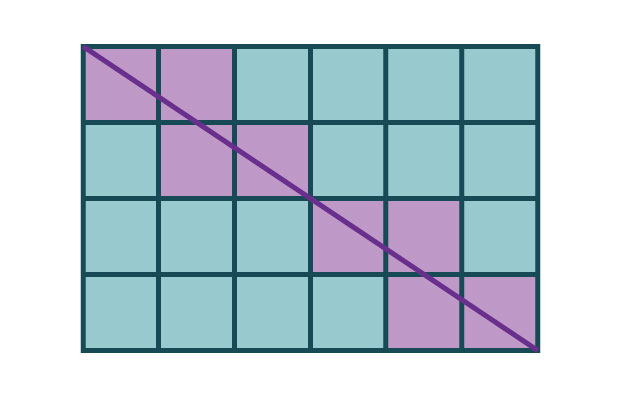# What's new## Diagonals on a grid

, 18 March 2020

Moein is messing about with some pieces of graph paper. He’s cut out some rectangles, and he’s ruling a line from the top left corner to the bottom right corner of each piece of paper. Then he plans to count the number of squares that each diagonal goes through.

Difficulty: Easy
First, Moein is going to try a grid that’s five squares wide and five squares tall. How many squares does the diagonal go through?

Difficulty: Tricky
The next grid is five squares wide and four squares tall. How many squares does the diagonal go through this time?

Difficulty: Taxing
Finally, there’s a grid that’s six squares wide and four squares tall. How many squares does this diagonal go through?

Scroll down or click for a hint, or the answer!## Brainteaser hint

It might help to try some smaller examples first.
• With a grid that’s two squares wide and two squares tall, the diagonal goes through two squares.
• With a grid that’s two squares wide and three squares tall, the diagonal goes through four squares.

If all else fails, you could get some graph paper and do it yourself!Five squares wide and five squares tall
The diagonal passes through the middle of the five squares on the diagonal of the grid! So the answer is five.Five squares wide and four squares tall
As the diagonal moves from one square to the next, it has three options.
• The line can go out the side of the square into a new column.
• The line go out the bottom of the square into a new row.
• The line go out the bottom corner of the square into a new column and row at the same time.
The diagonal goes into a new column four times and into a new row three times. Each time it goes through an edge. This line will not touch any corners except the one it starts on and the one it ends on. The diagonal starts in a square and crosses seven edges, which means it’ll touch eight squares in total.Six squares wide and four squares tall
With this grid, you can use the same strategy as the previous grid. But there’s one big difference. The diagonal will pass through the middle of the paper, which is a corner. This is the only corner it will go through.

The diagonal starts in a square and goes into a new column five times and a new row three times. If these were all edge crossings, that would make nine squares. But the diagonal crosses one row and column at the same time, so this line only visits eight squares!For more brainteasers and puzzles for kids, subscribe to Double Helix magazine!Categories:

## Similar posts

1.